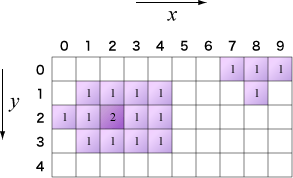Time Limit : sec, Memory Limit : KB

# Dropping Ink

As shown in the following figure, there is a paper consisting of a grid structure where each cell is indicated by (x, y) coordinate system.

We are going to put drops of ink on the paper. A drop comes in three different sizes: Large, Medium, and Small. From the point of fall, the ink sinks into surrounding cells as shown in the figure depending on its size. In the figure, a star denotes the point of fall and a circle denotes the surrounding cells.Originally, the paper is white that means for each cell the value of density is 0. The value of density is increased by 1 when the ink sinks into the corresponding cells. For example, if we put a drop of Small ink at (1, 2) and a drop of Medium ink at (3, 2), the ink will sink as shown in the following figure (left side):In the figure, density values of empty cells are 0. The ink sinking into out of the paper should be ignored as shown in the figure (top side). We can put several drops of ink at the same point.

Your task is to write a program which reads a sequence of points of fall (x, y) with its size (Small = 1, Medium = 2, Large = 3), and prints the number of cells whose density value is 0. The program must also print the maximum value of density.

You may assume that the paper always consists of 10 × 10, and 0 ≤ x < 10, 0 ≤ y < 10.

## Input

x1,y1,s1
x2,y2,s2
:
:


(xi, yi) represents the position of the i-th drop and si denotes its size. The number of drops is less than or equal to 50.

## Output

Print the number of cells whose density value is 0 in first line.
Print the maximum value of density in the second line.

## Sample Input

2,5,3
3,6,1
3,4,2
4,5,2
3,6,3
2,4,1


## Output for the Sample Input

77
5